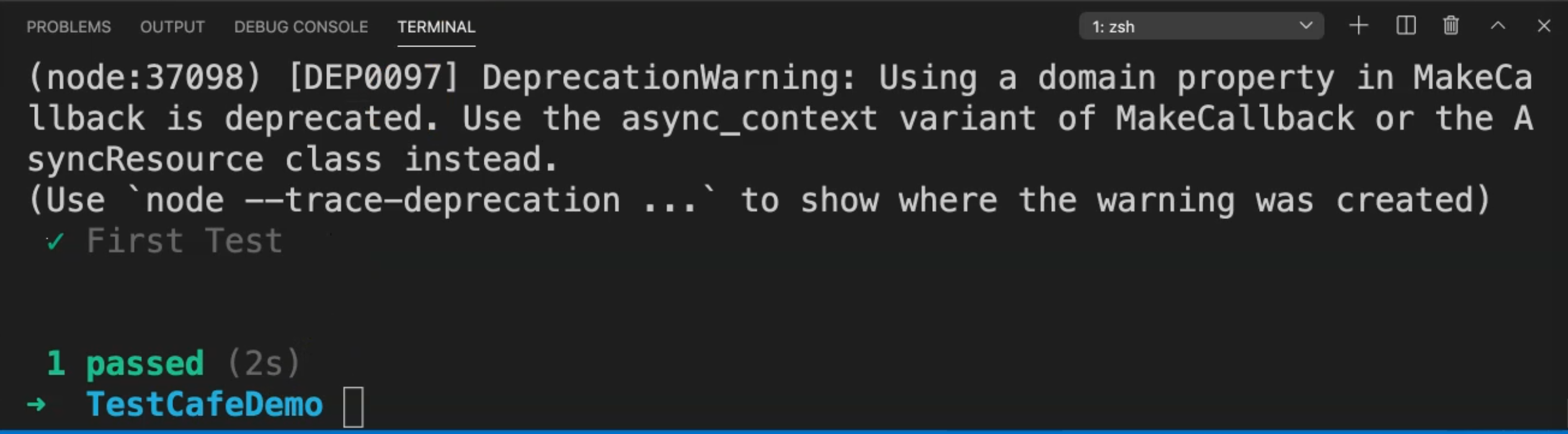Transcripted Summary

### # Using Selectors with Element

• The Selector API provides methods and properties to select elements on the page and get their state

• TestCafe uses standard CSS selectors to locate elements

• It's like when you use document.querySelector in JavaScript or FindElementBy.CSSSelector in Selenium Webdriver

So here, for example, if you wanted to write a text inside one element or text box, we need at the beginning to call `.typeText()`, and then we are passing here the CSS selector, and then we are adding the value that we want to enter or type in this text.

``````.typeText('#developer-name', 'John Smith');
``````

Or if you want to click on a button, here we are passing only the element with the CSS selector.

``````.click('#submit-button');
``````

In this demo, we will learn how to use selectors for finding elements using TestCafe and JavaScript. We will use a previous demo that we did, and the previous video was the first test. We will copy this class and we will rename it to `firstSelectorTest.js`.

After that, in our test, before the fixture, we need to import the selector from TestCafe. So we will use the `import` keyword. And after that, we can use arrays if we need to import different names.

So, here we will import the selector from TestCafe, and add a semicolon.

``````import {Selector} from 'testcafe';
``````

So here, we are importing the selectors TestCafe that we will be using instead of using the selectors as a hard-coded in our scripts. So we need to edit them, add the constants, and after that, we can use it.

So before the fixture, we will also add `const developerName` which is the Selector we imported and the selector will be `#developer-name`.

Then we will do the same for the rest of the elements.

So `const osOption`, for example, or the operating system option will be the Selector for #macos.

The last one is our button, so we can set `const submitButton` to the Selector for `#submit-button`.

So here we have the three selectors where we created constants for our selectors. Then we can replace the hard-coded values with a `const`.

``````import { Selector } from 'testcafe'

const developerName = Selector('#developer-name');
const osOption = Selector('#macos');
const submitButton = Selector('#submit-button');

fixture("First Fixture")
.page("https://devexpress.github.io/testcafe/example/");
test("First Test", async t => {
await t
.typeText(developerName,"TAU")
.click(osOption)
.click(submitButton);
});
``````

So here we are using constants and after that, we can use a constant if we for example, we need to assert any other values - if we need to use it in another call or another function, so we can use it in all the tests.

So here we replace the hardcoded values for the selectors with the selector function from TestCafe.

So let's run our test.

We will not run `firstTest.js`, but we will run the `firstSelectorTest.js` case to check that everything is working fine after we did the changes. So here we are opening the server, TestCafe would be redirected to the URL and our test passed.